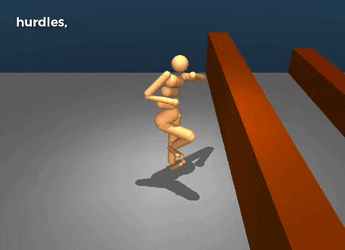# Markov Decision Process (MDP)Solutions:

Used where outcomes are partly random and partly under the control of a decision maker. MDP is a discrete time stochastic control process. At each time step, the process is in some state s, and the decision maker may choose any action a that is available in state s. The process responds at the next time step by randomly moving into a new state s', and giving the decision maker a corresponding reward R_{a}(s,s')} R_a(s,s'). The probability that the process moves into its new state s' is influenced by the chosen action. Helping the convergence of certain algorithms a discount rate (factor) makes an infinite sum finite.

## (Richard) Bellman Equation